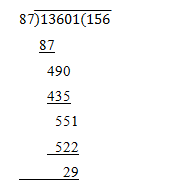# Number Systems ( 21 to 25 )

#### Prev     Next

Number Systems ( 21 to 25 ):

# APTITUDE QUESTIONS (NUMBER SYSTEMS):

21. If x and y are positive integers such that (6x + 3y) is a multiple of 11, then which of the following will be divisible by 11?

A)    4x + 6y

B)    2x + 4y

C)    8x + 3y

D)    3x – 4y

E)    None of these

Explanation:

By hit and trial, we put x = 5 and y = 1 so that (6x + 3y) = (6 * 5 + 3 * 1) = 33, which is divisible by 11

∴ (4x + 6y) = (4 * 5 + 6 * 1) = 26, which is not divisible by 11

(2x + 4y) = (2 * 5 + 4 * 1) = 14, which is not divisible by 11

(8x + 3y) = (8 * 5 + 3 * 1) = 43, which is not divisible by 11

(3x – 4y) = (3 * 5 – 4 * 1) = 11, which is divisible by 11

22. If 60% of 3/5 of a number is 36, then the number is:

A)    80

B)    100

C)    75

D)    90

E)    None of these

Explanation:

Let the number be x.

Then, 60% of 3/5 of x = 36

• 60/100 * 3/5 * x = 36
• x = (36 * 25/9) = 100

∴ required number = 100

23. How many of the following numbers are divisible by 132?

264, 396, 462, 792, 968, 2178, 5184, 6336

A)    7

B)    5

C)    4

D)    8

E)    None of these

Explanation:

132 = 4 * 3 * 11

264 = 4, 3, 11

396 = 4, 3, 11

792 = 11, 3, 4

462 = 11,3

968 = 11, 4

2178 = 11, 3

5184 = 3, 4

6336 = 11, 3, 4

Required Numbers are = 4

24. If n is natural number, then (6n2 + 6n) is always divisible by:

A)    6 only

B)    6 and 12 both

C)    12 only

D)    18 only

E)    None of these

Explanation:

(6n2 + 6n) = 6n(n + 1), which is always divisible by 6 and 12 both, since n(n + 1) is always even.

25. What least number must be subtracted from 13601, so that the remainder is divisible by 87?

A)    33

B)    23

C)    29

D)    35

E)    None of these

Explanation:Required Number = 29